##### Trigonometry Workbook For DummiesAlthough factoring quadratics is a breeze, factoring trigonometry equations with higher degrees can get a bit nasty if you don’t have a nice situation such as just two terms or a quadratic-like equation. Below, you see two such problems: 2sin3 x = sin x and 2cos4 x – 9cos2 x + 4 = 0.

The first equation has just two terms, so you can factor it by finding a greatest common factor. Solve 2sin3 x = sin x for all the possible angles in degrees.

1. Move the term on the right to the left by subtracting it from each side.

2sin3 x – sin x = 0

2. Factor out sin x.

sin x (2sin2 x – 1 = 0)

3. Set each factor equal to 0.

sin x = 0 or 2sin2 x – 1 = 0

4. Solve the two equations for the values of x that satisfy them.

If sin x = 0, then x = sin–1(0) = 0°, 180°, . . . or 0° + 180°n.

If 2sin2 x – 1 = 0, 2sin2 x = 1, sin2 x = 1/2, then you end up with a quadratic equation.

5. Take the square root of both sides of the quadratic equation and solve for x.

Multiply both parts of the fraction by the denominator to get the radical out of the denominator.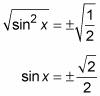Now, considering both solutions: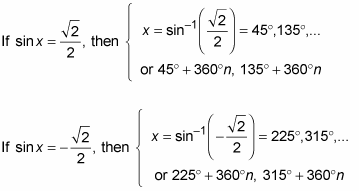This fourth-degree trig equation has a whole slew of answers:

x = 180°n

x = 45° + 360°n

x = 135° + 360°n

x = 225° + 360°n

x = 315° + 360°n

You can combine these last four equations for x, the ones that begin with multiples of 45 degrees, to read x = 45° + 90°n. This equation generates all the same angles as the last four statements combined. How do you know you can simplify this way? Because the angles of 45, 135, 225, and 315 degrees are all 90 degrees apart in value. By starting with the 45 and adding 90 over and over, you get all the listed angles as well as the infinite number of their multiples.

The next example is also a fourth-degree equation, but this one is quadratic-like, meaning that it factors like a quadratic trinomial into two binomial factors. This problem has the possibility of having a great number of solutions — or none. Solve 2cos4 x – 9cos2 x + 4 = 0 for the solutions that are between 0 and 2π.

1. Factor the trinomial as the product of two binomials.

(2cos2 x – 1)(cos2 x – 4) = 0

2. Set each factor equal to 0.

2cos2 x – 1 = 0 or cos2 x – 4 = 0

3. Solve for the function in each equation by getting the terms with cosine in them alone on one side of the equation.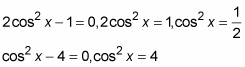4. Take the square root of each side of each equation.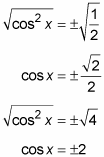5. Solve for the values of x that satisfy the equations.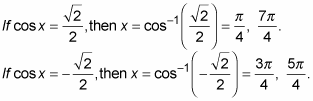If cos x = ±2, then you have a problem — that equation doesn’t compute! The cosine function results only in values between –1 and 1. This factor doesn’t give any new solutions to the original problem.